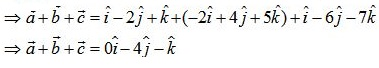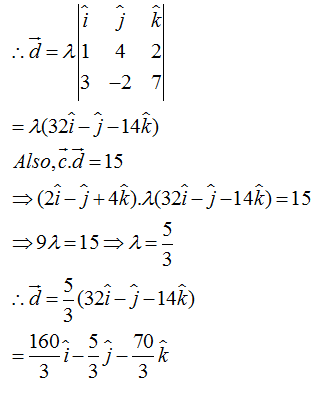# Topic: Vector Algebra (Test 1)

Topic: Vector Algebra
Q.1
Ifare  two collinear vectors, then which of the following are incorrect
A. both the vectorshave same direction, but different magnitudes.
B. the respective components ofare not proportional
C.D.Answer : Option A
Explaination / Solution:

Ifare two collinear vectors, then, they are parallel to the same line irrespective of their magnitudes and directions.

Workspace
Report
Q.2
Magnitude of the vectoris
A. √63
B. √61
C. √62
D. √65
Answer : Option C
Explaination / Solution:Workspace
Report
Q.3
Magnitude of the vectoris
A. √3
B. 1
C. 0.5
D. 1.5
Answer : Option B
Explaination / Solution:Workspace
Report
Q.4
Find the scalar and vector components of the vector with initial point (2, 1) and terminal point (– 5, 7).
A.B.C. -7 and 6; -D.Answer : Option C
Explaination / Solution:

The scalar and vector components of the vector with initial point (2, 1) and terminal point (– 5, 7) is given by : (- 5 – 2 ) i.e. – 7 and (7 – 1 ) i.e. 6. Therefore, the scalar components are – 7 and 6 .,and vector components are -Workspace
Report
Q.5

Find the values of x and y so that the vectors  are equal

A. x = 3, y = 2
B. x = 3, y = 2
C. x = 2, y = 3
D. x = 3, y = 3
Answer : Option C
Explaination / Solution:

x = 2, y = 3

Workspace
Report
Q.6
Find the sum of the vectorsA. i^4j^k^
B. i^+4j^k^
C. i4j^k^
D. i^4j^k^
Answer : Option C
Explaination / Solution:

We have:
vectorsWorkspace
Report
Q.7
Write down a unit vector in XY-plane, making an angle of 30° with the positive direction of x-axis.
A. 32i^12j^
B. 32i^12j^
C. 32i^+12j^
D. 32i^+12j^
Answer : Option D
Explaination / Solution:

Let be a unit vector in XY plane,making angle 300 with positive X axis,so we have the vector as

is the required vector.

Workspace
Report
Q.8
Find the value of x for which is a unit vector
A. ±12
B. ±17
C. ±15
D. ±13
Answer : Option D
Explaination / Solution:

As x is a unit vector ,
therefore,

Workspace
Report
Q.9
Find the position vector of a point R which divides the line joining two points P and Q whose position vectors areexternally in the ratio 1 : 2. Also, show that P is the mid point of the line segment RQ.
A.B.C.D.Answer : Option C
Explaination / Solution:Workspace
Report
Q.10A. 13(160i^5j^+70k^)
B. 13(160i^5j^+70k^)
C. 13(160i^5j^-70k^)
D. 13(160i^+5j^70k^)
Answer : Option C
Explaination / Solution:

LetWorkspace
Report